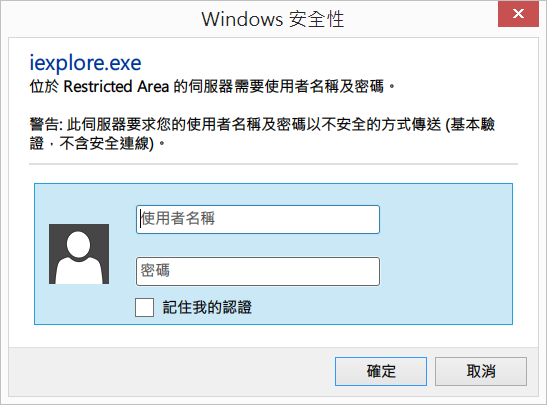## Archive for the ‘ Website ’ Category

### [轉載][PHP]正規表示式基本語法

• ^The：表示所有以「The」開始的字串（「There」、「The cat」等）；
• of despair\$：表示所有以「of despair」結尾的字串；
• ^abc\$：表示開始和結尾都是「abc」的字串--呵呵，只有「abc」自己了；
• notice：表示任何包含「notice」的字串。

### Everything is Object in JavaScript

```alert (typeof('abc'));
var ary = [123, "abc"];

### Create Objects in JavaScript

```var main = new Object;     // 建立新物件
main.x = 123; // 設定物件成員變數(屬性)之一
main["y"] = "XYZ"; // 設定物件成員變數(屬性)之二

### List All Members in an Object

```function listMember(main) {
var s = "";
for( key in main )  // 使用 in 運算子列舉所有成員
s += key + ": " + main[key] + "\n";
return s;
}```

```var ary = [123, "abc"];

### Construct Object with Initial Value

```function Array2DVar(x,y) {  // 定義二維陣列原型
this.length = x;
this.x = x;  // x 維度長度
this.y = y;  // y 維度長度
for(var i = 0; i < this.length; i++)  // 初始各元素值為 null
this[i] = new Array(y);  // this 代表物件本身
}

var a2dv = new Array2DVar(10, 10);  // 建立新的 10*10 的二維陣列
a2dv = "ABC";  // 設定二維陣列元素值
a2dv = "XYZ";
a2dv = 1000;

### Initial Array Object

```a = new Array("abc", "xyz", 1000); // constructor 語法，或
a = ["abc", "xyz", 1000]; // 陣列標準語法```

```b = [ // 使用函式作為陣列元素
];

for (var i = 0; i < b.length ; i++)
b[i]();```

### Object as Association Array

```obj1 = {"a" : "Athens" , "b" : "Belgrade", "c" : "Cairo"};
obj2 = {
name: "Edward",
showName: function() { alert(this.name); }  // 使用函式作為物件屬性
}
obj2.showName();  // 顯示 Edward
obj2.age = 23;  // 屬性可以動態加入```

### Object as Return Value

```function a () {
return [32, 17];
}
b = a();
function pixel () {
return {"x": 32, "y":17};
}
point = pixel ();
alert (point.x + "\n" + point.y);  // 或
alert (pixel().x + "\n" + pixel().y);```

### Delegation Function Object

```function doloop(begin, end, func) {  // 這個函式是個 iterator
for (var i = begin; i < end; i++) {
func(i);
}
}
function func1(i) {  // 印出 ** n **
document.writeln("** " + i + " **<br>");
}
doloop(1, 10, func1);  // 印出 10 行 ** n **
doloop(20, 29,
function(i) {  // 印出 10 行 ## n ##
document.writeln("## " + i + " ##<br>");
}
);```

### Object = Properties + Behaviors

```function Array2D(x,y){  // 以一維陣列模擬二維陣列的原型物件
this.length = x * y;  // 陣列總長
this.x = x;  // x 維度長度
this.y = y;  // y 維度長度
for(var i = 0; i < this.length; i++)  // 初始各元素值為 null
this[i] = null;
this.get = function(x,y){  // 成員函式：取得陣列第 [x,y]個元素值
return this[x*this.x + y];
}
this.set = function(x,y,value){  // 成員函式：設定陣列第 [x,y] 個元素值
this[x*this.x + y] = value;
}
}```

```var a2d = new Array2D(10, 10);  // 建立新的「二維」陣列

a2d.set(1, 3, "ABC");  // 設定「二維」陣列元素值
a2d.set(2, 6, "XYZ");
a2d.set(9, 9, 1000);

### Member Function Outside of Constructor

```function Array2D(x,y){  // 以一維陣列模擬二維陣列的原型物件
this.length = x * y;  // 陣列總長
this.x = x;  // x 維度長度
this.y = y;  // y 維度長度
for(var i = 0; i < this.length; i++)  // 初始各元素值為 null
this[i] = null;
this.get = Array2DGet;  // 用這種方式把成員函式掛進來
this.set = Array2DSet;
}

function Array2DGet(x,y){  // 成員函式：取得陣列第 [x,y] 個元素值
return this[x*this.x + y];
}

function Array2DSet(x,y,value){  // 成員函式：設定陣列第 [x,y] 個元素值
this[x*this.x + y] = value;
}```

### Dynamic Object Function

```function array_max(){  // 定義求取 Array 最大值之函式
var i, max = this;
for (i = 1; i < this.length; i++){
if (max < this[i])
max = this[i];
}
return max;
}
Array.prototype.max = array_max;  // 在 Array 原型中加入 max 函式```

```var x = new Array(1, 2, 3, 4, 5, 6);  // 透過 Array 建構子建立一陣列，想求取 x 中某一元素之最大值
var y = x.max( );  // 取得 x 之最大元素```

### Dynamic Mix in

```function main(){  // main 之建構子
// ...
this.imports =
function (object) {
if( typeof object == "object")
for( value in object )
this[value] = object[value];
}
// ...
}
obj = new main();
main.imports(new dynamic());  // 匯入 dynamic 物件之所有功能```

### .htaccess 簡易的網頁帳號認證

.htaccess 讓你不用寫程式，就可以用最簡單的方式做出「網頁登入」的功能，限制哪些用戶能進到網頁的某一層目錄。

## 建立用戶驗證資料

### 建立用戶驗證檔

`htpasswd -c /path/to/.htusers UserName1`

`htpasswd /path/to/.htusers UserName2`

### 建立群組資料檔

`Group1:UserName1 UserName2`

## 驗證登入

### 針對用戶:

```AuthName "Restricted Area" # 名稱必須用英文
AuthType Basic
AuthUserFile /path/to/.htusers

`require valid-user # 針對全部用戶`

### 針對群組:

```AuthName "Restricted Area" # 名稱必須用英文
AuthType Basic
AuthUserFile /path/to/.htusers
AuthGroupFile /path/to/.htgroups
require group Group1 # 僅針對特定群組的用戶，以空白分隔多個群組```

### 針對特定檔案:

```<Files FileName>
require valid-user
</Files>```

<Files>~</Files> 一次只能填寫一個檔案，但支援正規表示式。以下是使用正規表示式限制圖檔存取的範例:

```<Files ~ "\.(gif|jpe?g|png)\$">
require valid-user
</Files>```

```<FilesMatch "\.(gif|jpe?g|png)\$">
require valid-user
</FilesMatch>```` http://UserName:Password@www.example.com/directory/`

## 問題排除

`AllowOverride All`

## 參考網頁

### [轉載]Unicode 脫逸序列轉換

```<META HTTP-EQUIV="Content-Type" CONTENT="text/html; charset=big5">
<script language=javascript>
<!--
function StringToUniCode(source) {
sret = "";
// Unicode 跳脫序列之格式為 \u00ff
for(var i=0; i < source.length; i++) {
var num = new Number( source.charCodeAt(i));
sret += "\\u" + num.toString(16);
}
return sret;
}
function convert(){
document.forms.content.result.value = StringToUniCode(document.forms.content.source.value);
}
//-->
</script>

<form name=content>

<input type=button value=轉換 onclick="convert()">
</form>```

### HTTP 回應的代碼含義

HTTP 的回應代碼是三位數字，其中第一個數字定義了代碼的類別:

100～199 訊息 請求被接收，正在處理
200～299 成功 動作被成功接收、理解和接受
300～399 重新導向 必須採取其他動作才能完成請求
400～499 用戶錯誤 請求包含不良語法或無法完成
500～599 伺服器錯誤 伺服器無法處理的請求

• 200: 請求成功
• 400: 語法錯誤
• 401: 未經授權
• 403: 禁止訪問
• 404: 請求的網頁不存在
• 500: 伺服器錯誤